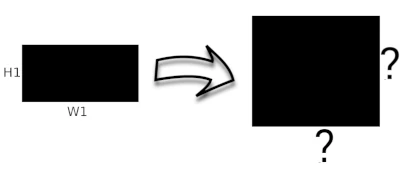# Aspect Ratio Calculator

## Simple Aspect Ratio Calculator

In order to find the aspect ratio of an image or video, you need the width and height of the image or video. Just insert them into the calculator below to find the aspect ratio.

Alternatively you can choose one of the common ratios in the dropdown menu below and it will automatically give you a sample resolution:

Common aspect ratios (Optional):

### H1 - Height:

Result:

Aspect Ratio (in decimals):

Aspect Ratio (Closest full fraction):

## Finding the new height or width

Use this tool if you know the aspect ratio and need to find the new width (or height) of the image or video.

Just insert one of them into the calculator below to find the other value (That also respects the aspect ratio selected above).### H2 - Height:

Result:

Your new image or video should have (in Pixels):

X(Pixels)

Width:

Height: